# SVMs¶

Note

On the homework:

$\begin{split}& P(GPA=x|type=N) \\ & = \frac{1}{\sqrt{2\pi \sigma_N^2}} \exp(\frac{-(x-\mu_N)^2}{2\sigma_N^2})\end{split}$

How do we estimate $$\mu$$ and $$\sigma$$ from the data?

1. $$\arg \max_{\mu, \sigma} P(GPA1, GPA2..GPA6|\mu, \sigma)$$
2. $$\hat{\mu}_N = avg(GPA)$$

Support Vector Machines

## Max-Margin Classification¶

This is a linearly separable dataset, and all these hyperplanes are valid, but which one is best?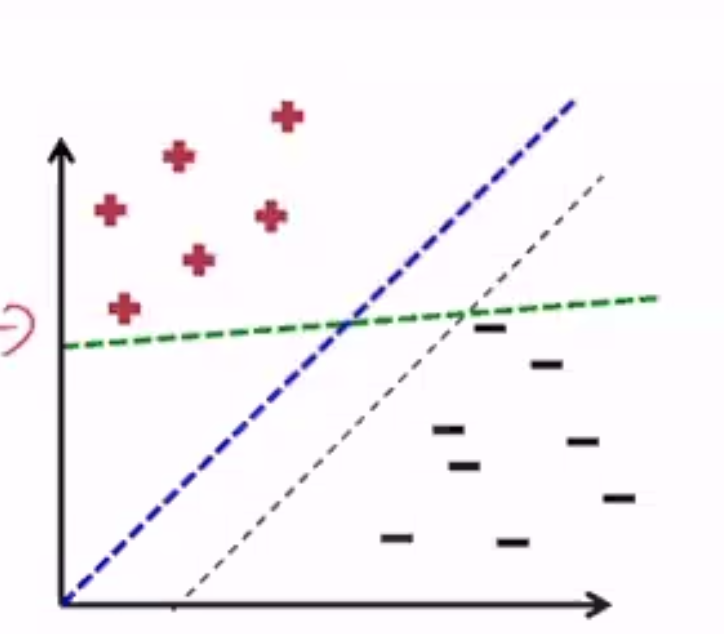• The blue one has the largest margin
• Margin: Distance between the hyperplane and the nearest point
• defined for a given dataset $$\mathbf{D}$$ and hyperplane $$(\mathbf{w}, b)$$
$\begin{split}margin(\mathbf{D}, \mathbf{w}, b) = & \min_{(x, y)\in \mathbf{D}} y(\mathbf{w \cdot x} + b) & \text{ if w separates D} \\ & -\inf & \text{ otherwise}\end{split}$

SVM is a classification algorithm that tries to find the maximum margin separating hyperplane.

## Hard SVM¶

### Setup¶

• Input: training set of pairs $$<x_n, y_n>$$
• $$x_n$$ is the D-dimensional feature vector
• $$y_n$$ is the label - assume binary $$\{+1, -1\}$$
• Hypothesis class: set of all hyperplanes H
• Output: $$w$$ and $$b$$ of the maximum hypotheses $$h\in H$$
• $$w$$ is a D-dimensional vector (1 for each feature)
• $$b$$ is a scalar

### Prediction¶

• learned boundary is the maximum-margin hyperplane specified by $$w, b$$
• given a test instance $$x'$$, prediction $$\hat{y} = sign(w \cdot x' + b)$$
• if the prediction is correct, $$\hat{y}(w \cdot x' + b) > 0$$

### Intuition¶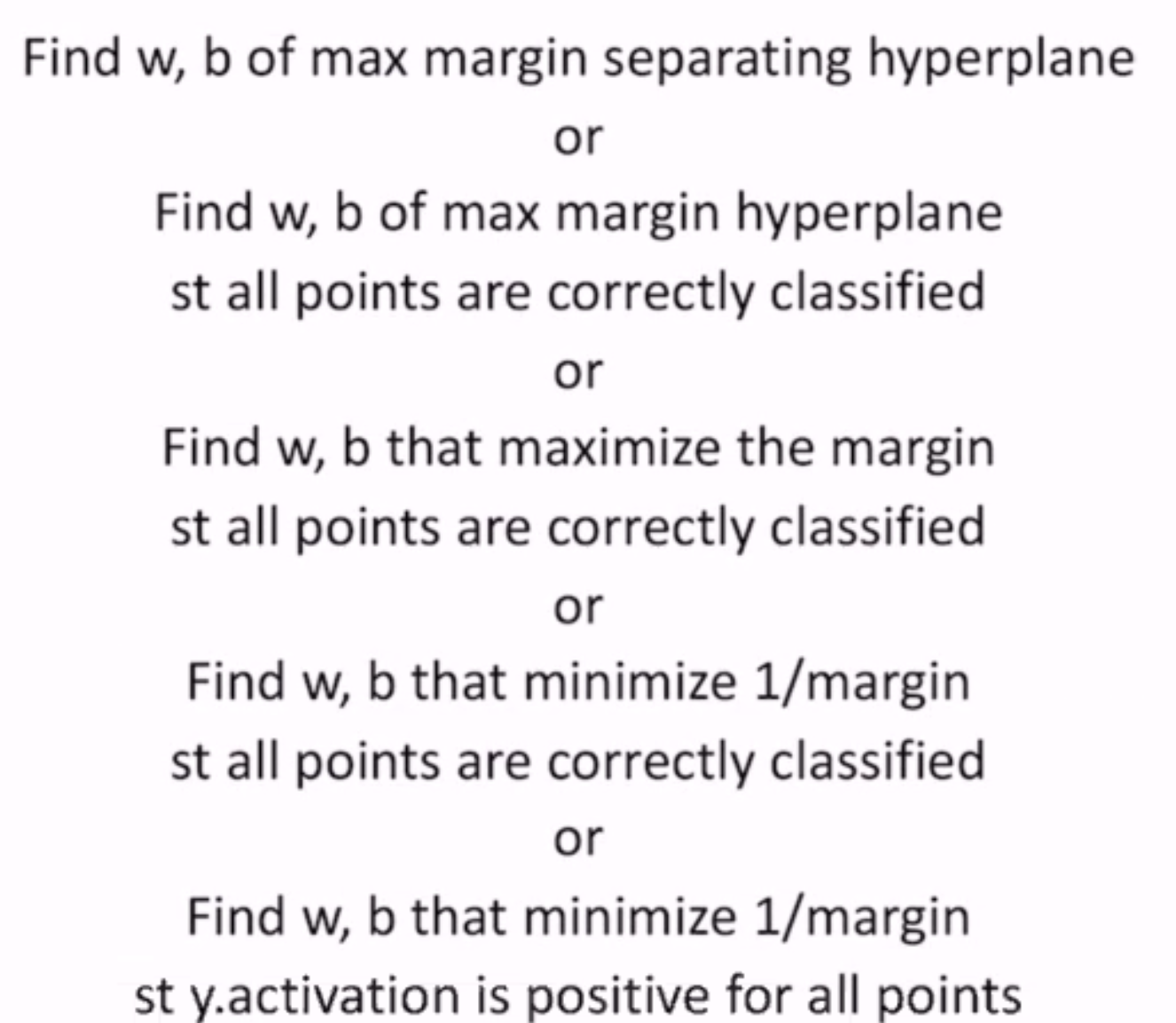But $$y * activation$$ is a weak condition - let’s increase it to be “sufficiently” positive

Final Goal: Find w, b that minimize 1/margin s.t. y * activation >= 1 for all points

### Optimization¶

$\begin{split}\min_{w, b} & \frac{1}{\gamma(w, b)} \\ \text{subj. to } & y_n(w \cdot x_n + b) \geq 1 (\forall n)\end{split}$

Where $$\gamma$$ is the distance from the hyperplane to the nearest point

• maximizing $$\gamma$$ = minimizing $$1/\gamma$$
• constraints: all training instances are correctly classified
• we have a 1 instead of 0 in the condition to ensure a non-trivial margin
• this is a hard constraint, and so called a hard-margin SVM
• what about for non linearly-separable data?
• infeasible solution (feasible set is empty): no hyperplane tielded
• let’s loosen the constraint slightly

## Soft-Margin SVMs¶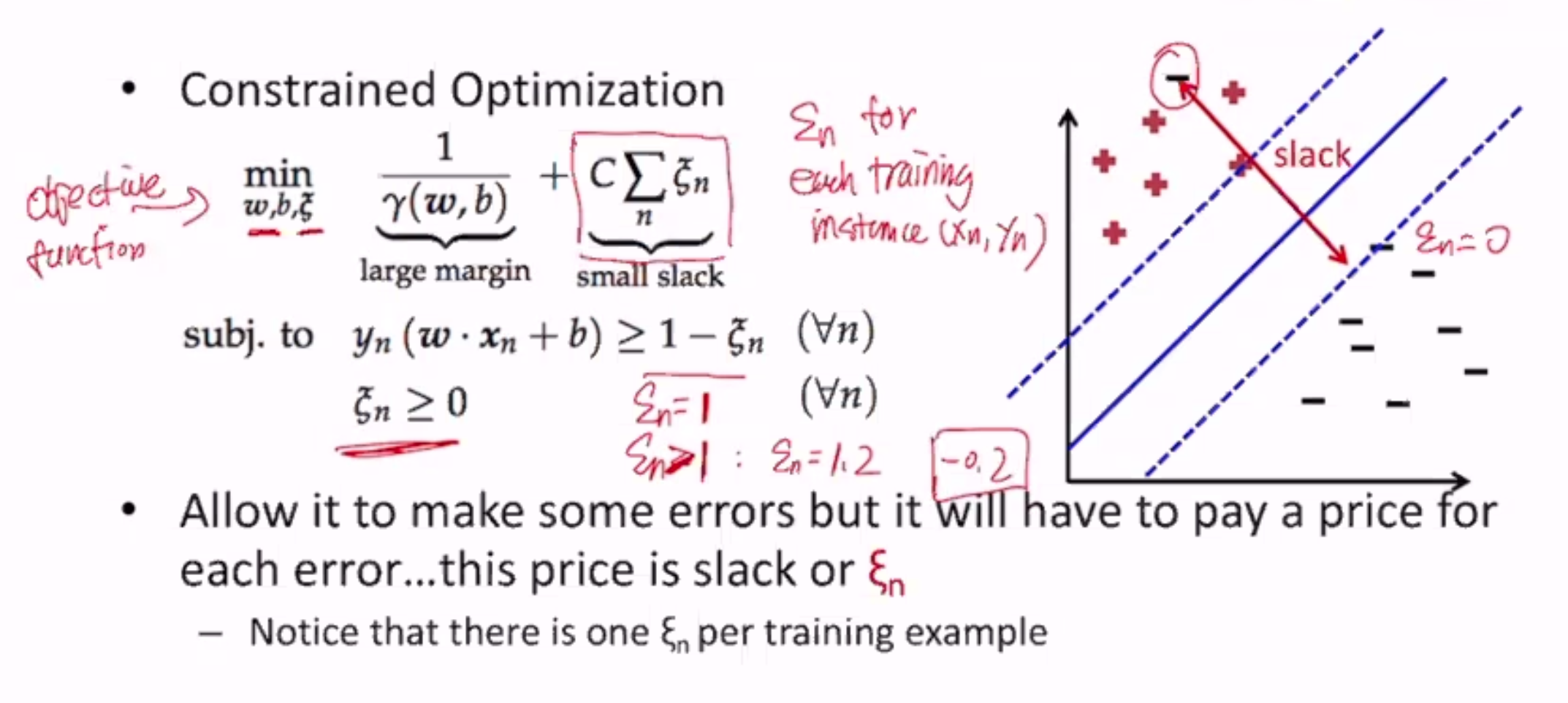• introduce one slack variable $$\xi_n$$ for each training instance
• if a training instance is classified correctly, $$\xi_n$$ is 0 since it needs no slack
• but $$\xi_n$$ can even be >1 for incorrectly classified instances
• if $$\xi_n$$ is 0, classification is correct
• if $$0 < \xi_n < 1$$, classification is correct but margin is not large enough
• if $$\xi_n > 1$$, classification is incorrect
• where $$C$$ is a hyperparameter (how much to care about slack)
• if the slack component of the objective function is 0, it’s the same goal as a hard-margin SVM

TLDR: maximize margin while minimizing total cost the model has to pay for misclassification that happens while obtaining this margin

## Discussion¶

Note that the max-margin hyperplane lies in the middle between the positive and negative points

• So the margin is determined by only 2 data points, that lie on the lines $$w \cdot x + b = 1$$ and $$w \cdot x + b = -1$$
• these points, $$x_+$$ and $$x_-$$, are called support vectors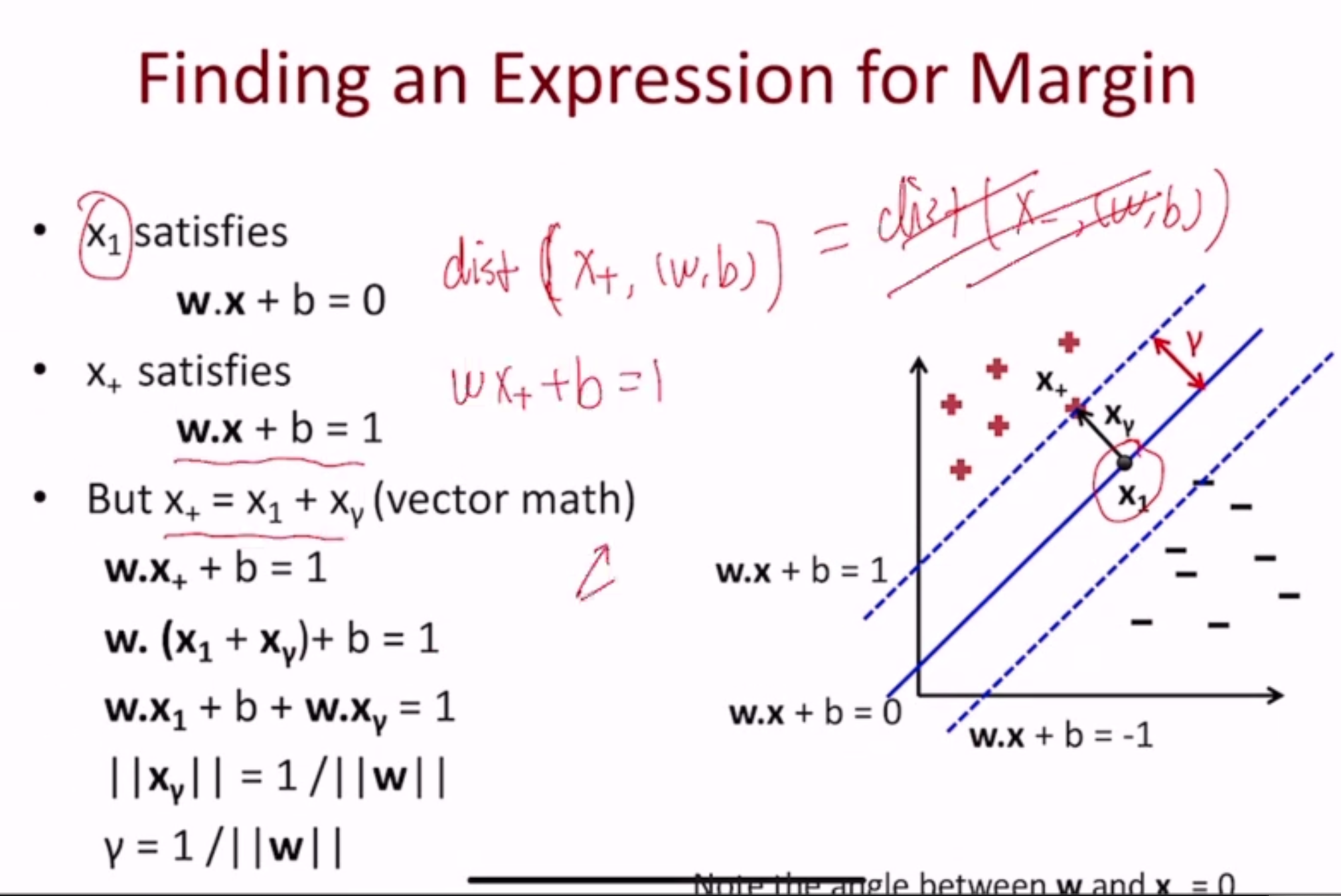Note

$$w \cdot x_1 + b$$ is 0 since $$x_1$$ is on the decision boundary

$$w \cdot x_\gamma = 1$$ -> $$||w||*||x_\gamma|| = 1$$ since $$w, x_\gamma$$ are parallel

Therefore, we can modify the objective:

$\begin{split}\min_{w, b, \xi} & \frac{1}{2}||w||^2 + C\sum_n \xi_n & \\ \text{subj. to } & y_n(w \cdot x_n + b) \geq 1 - \xi_n & (\forall n) \\ & \xi_n \geq 0 & (\forall n)\end{split}$

Or, intuitively, finding the smallest weights possible.

## Hinge Loss¶

We can write the slack variables in terms of $$(w, b)$$:

$\begin{split}\xi_n = & 0 & \text{ if } y_n(w\cdot x_n + b) \geq 1 \\ & 1 - y_n(w\cdot x_n + b) & \text{ otherwise}\end{split}$

which is hinge loss! Now, the SVM objective becomes:

$\min_{w, b} \frac{1}{2}||w||^2 + C\sum_n l^{(hin)}(y_n, w\cdot x_n + b)$

## Solving¶

### Hard-Margin SVM¶

$\begin{split}\min_{w, b} & \frac{1}{2}||w||^2 \\ \text{subj. to } & y_n(w \cdot x_n + b) \geq 1 (\forall n)\end{split}$
• convex optimization problem
• specifically a quadratic programming problem
• minimizing a function that is quadratic in vars
• constraints are linear
• this is called the primal form, but most people solve the dual form

We can encode the primal form algebraically:

$\min_{w, b} \max_{\alpha \geq 0} \frac{1}{2}(w \cdot w) + \sum_i \alpha_i (1-y_i(w \cdot x_i + b))$

### Dual Form¶

• does not change the solution
• introduces new variables $$\alpha_n$$ for each training instance
$\begin{split}\max & \sum_{n=1}^N \alpha_n - \frac{1}{2} \sum_{m,n=1}^N \alpha_m \alpha_n y_m y_n (x_m^T x_n) \\ \text{subject to } & \sum_{n=1}^N \alpha_n y_n = 0, \alpha_n \geq 0; n = 1..N\end{split}$

Once the $$\alpha_n$$ are computed, w and b can be computed as:

$\begin{split}w = \sum_{n=1}^N \alpha_n y_n x_n \\ b = \text{something...}\end{split}$

As it turns out, most $$\alpha_i$$’s are 0 - only the support vectors are not

For Soft-Margin SVM

$\begin{split}\max & \sum_{n=1}^N \alpha_n - \frac{1}{2} \sum_{m,n=1}^N \alpha_m \alpha_n y_m y_n (x_m^T x_n) \\ \text{subject to } & \sum_{n=1}^N \alpha_n y_n = 0, 0 \leq \alpha_n \leq C; n = 1..N\end{split}$

For soft-margin SVMs, support vectors are:

• points on the margin boundaries ($$\xi = 0$$)
• points in the margin region ($$0 < \xi < 1$$)
• points on the wrong side of the hyperplane ($$\xi \geq 1$$)

Conclusion: w and b only depend on the support vectors

#### Derivation¶

Given the algebriaecally encoded primal form:

$\min_{w, b} \max_{\alpha \geq 0} \frac{1}{2}(w \cdot w) + \sum_i \alpha_i (1-y_i(w \cdot x_i + b))$

We can switch the order of the min and max:

$\begin{split}\max_{\alpha \geq 0} \min_{w, b} \frac{1}{2}(w \cdot w) + \sum_i \alpha_i (1-y_i(w \cdot x_i + b)) \\ = \max_{\alpha \geq 0} \min_{w, b} L(w, b, \alpha)\end{split}$

To solve inner min, differentiate L wrt w and b:

$\begin{split}\frac{\partial L(w, b, \alpha)}{\partial w_k} & = w_k - \sum_i \alpha_i y_i x_{i,k} & \\ \frac{\partial L(w, b, \alpha)}{\partial w} & = w - \sum_i \alpha_i y_i x_i & \to w = \sum_i \alpha_i y_i x_i \\ \frac{\partial L(w, b, \alpha)}{\partial b} & = - \sum_i \alpha_i y_i & \to \sum_i \alpha_i y_i = 0\end{split}$
• $$w = \sum_i \alpha_i y_i x_i$$ means w is a weighted sum of examples
• $$\sum_i \alpha_i y_i = 0$$ means positive and negative examples have the same weight
• $$\alpha_i > 0$$ only when $$x_i$$ is a support vector, so w is a sum of signed support vectors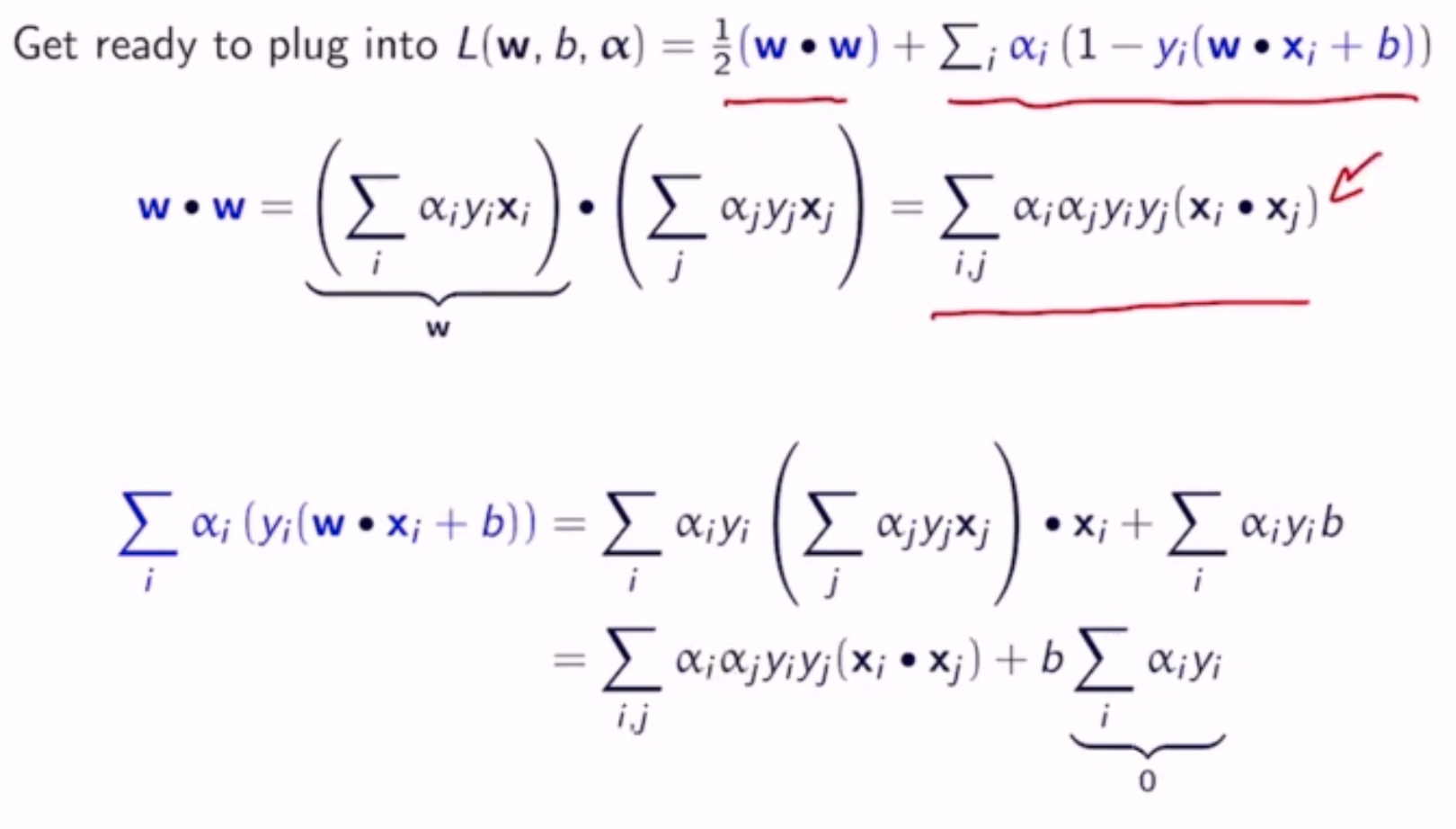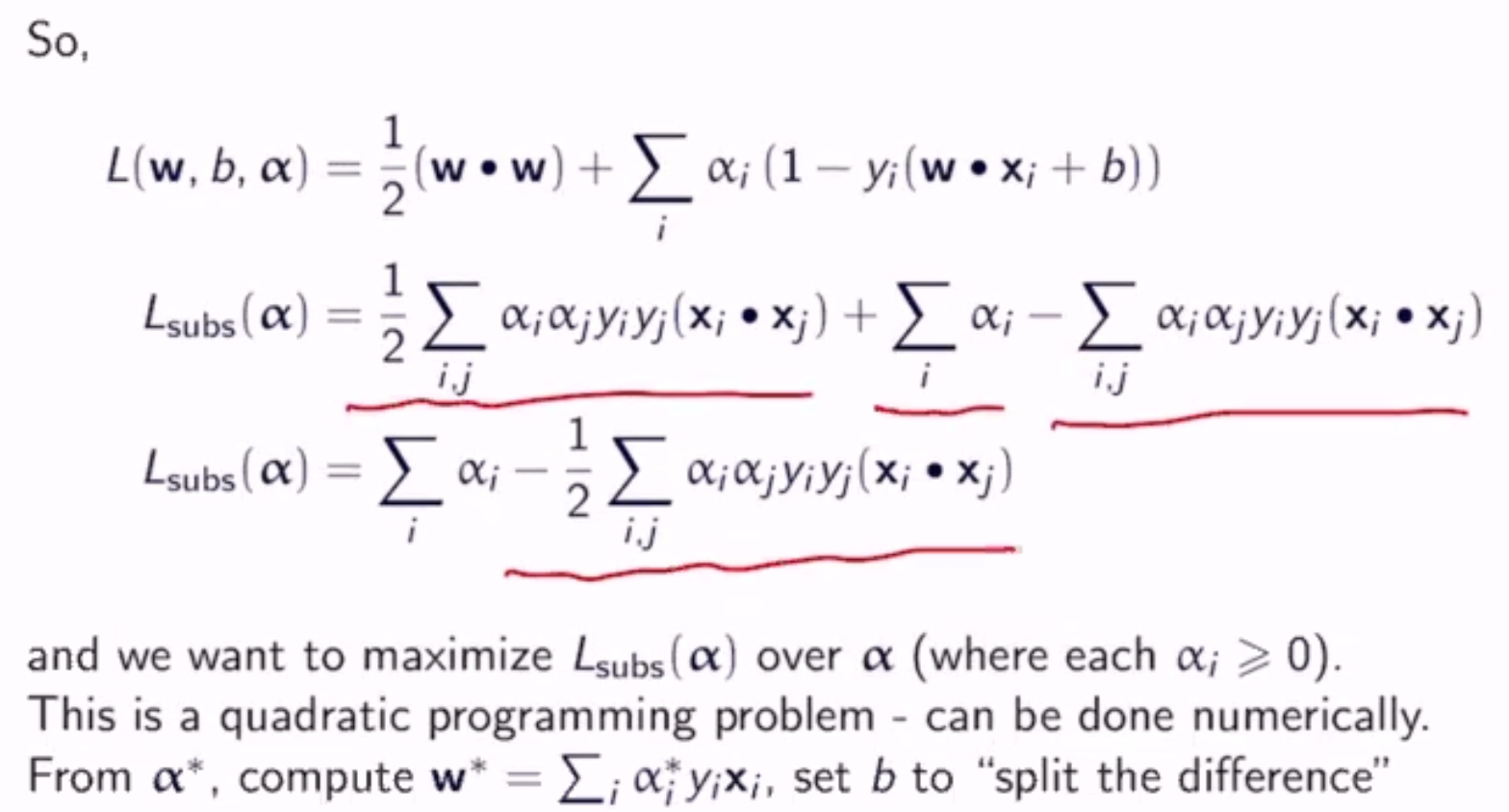Conclusion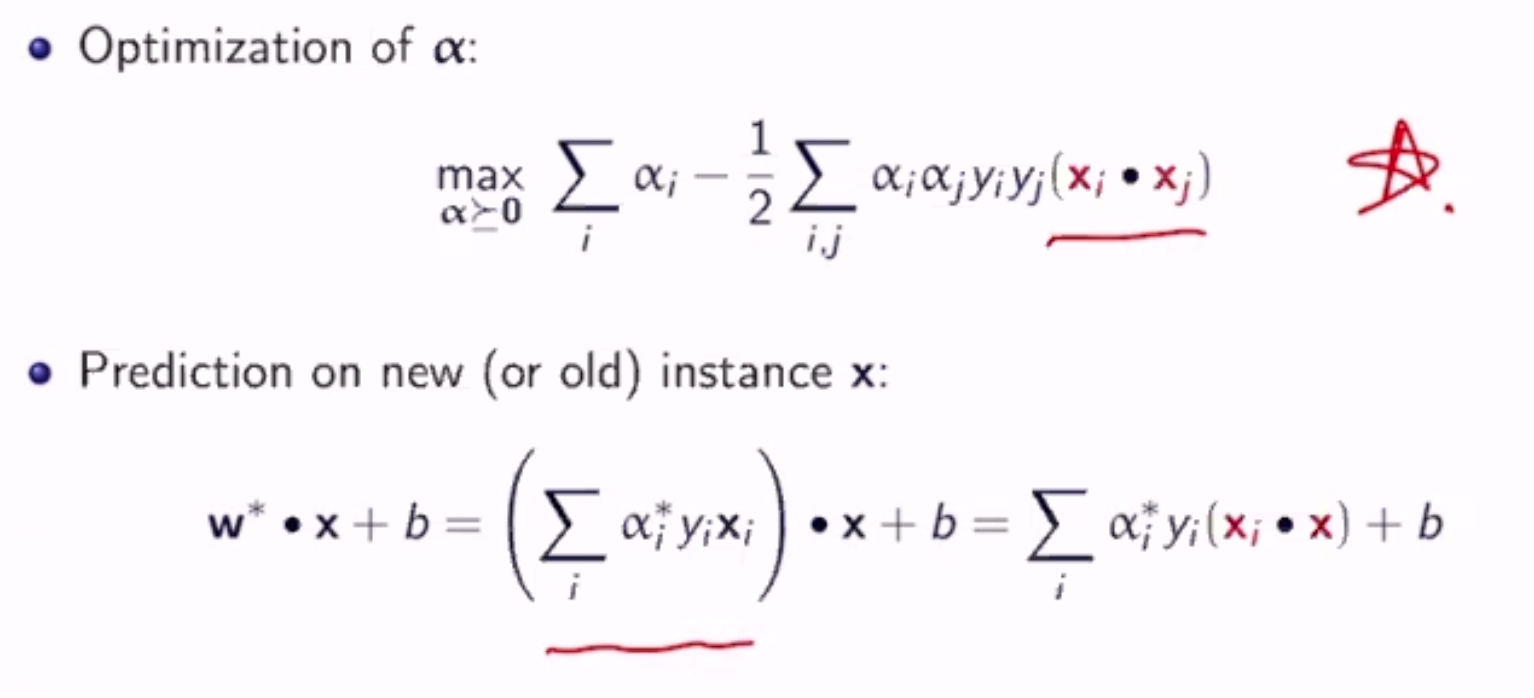Soft-Margin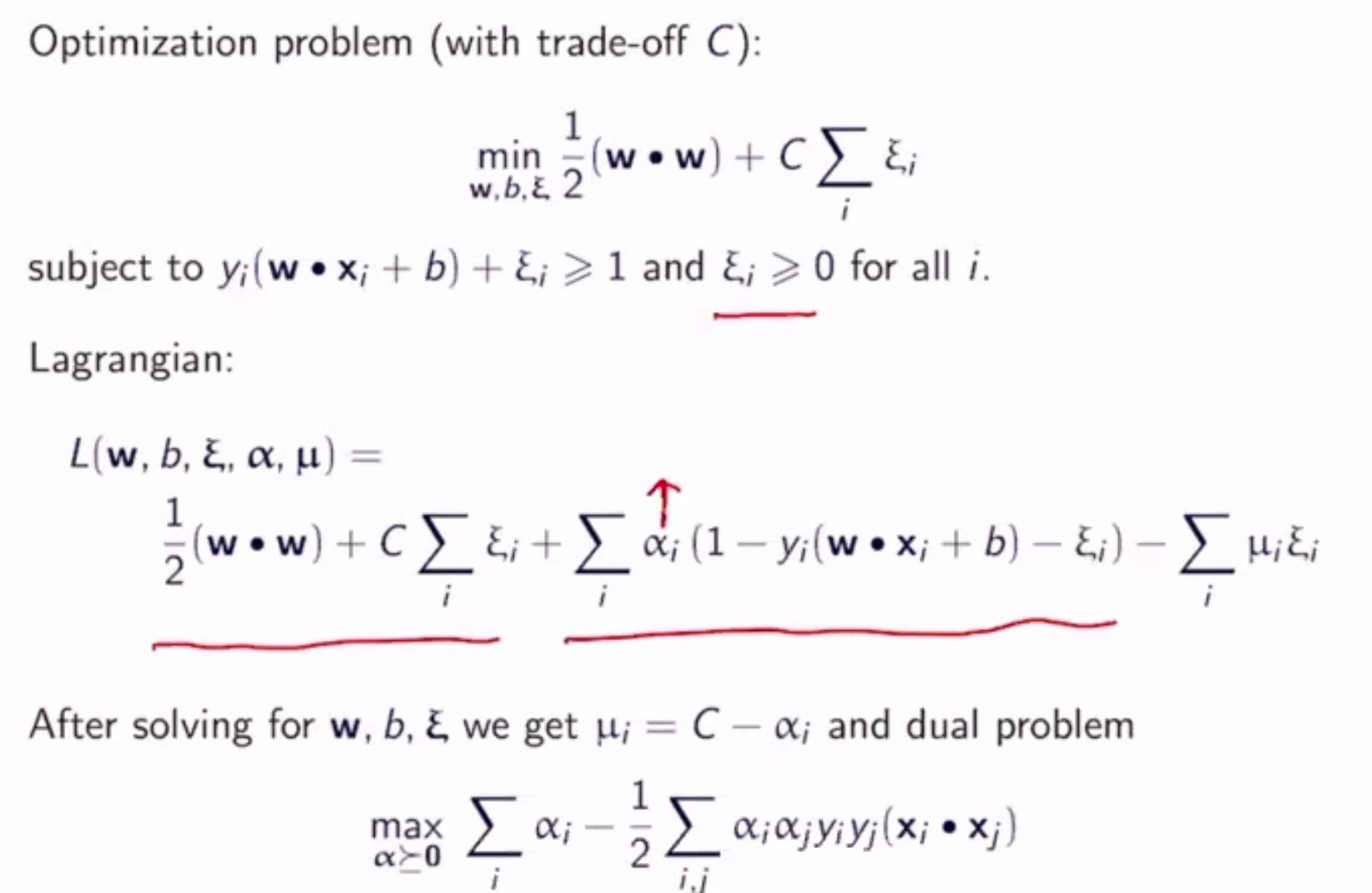subject to $$0 \leq \alpha_i \leq c$$ $$(\forall i)$$

## Non-Linearly-Seperable¶

What if our data is not linearly seperable?

• use a non-linear classifier
• transform our data so that it is, somehow
• e.g. adding a dummy dimension based on a quadratic formula of the real dimension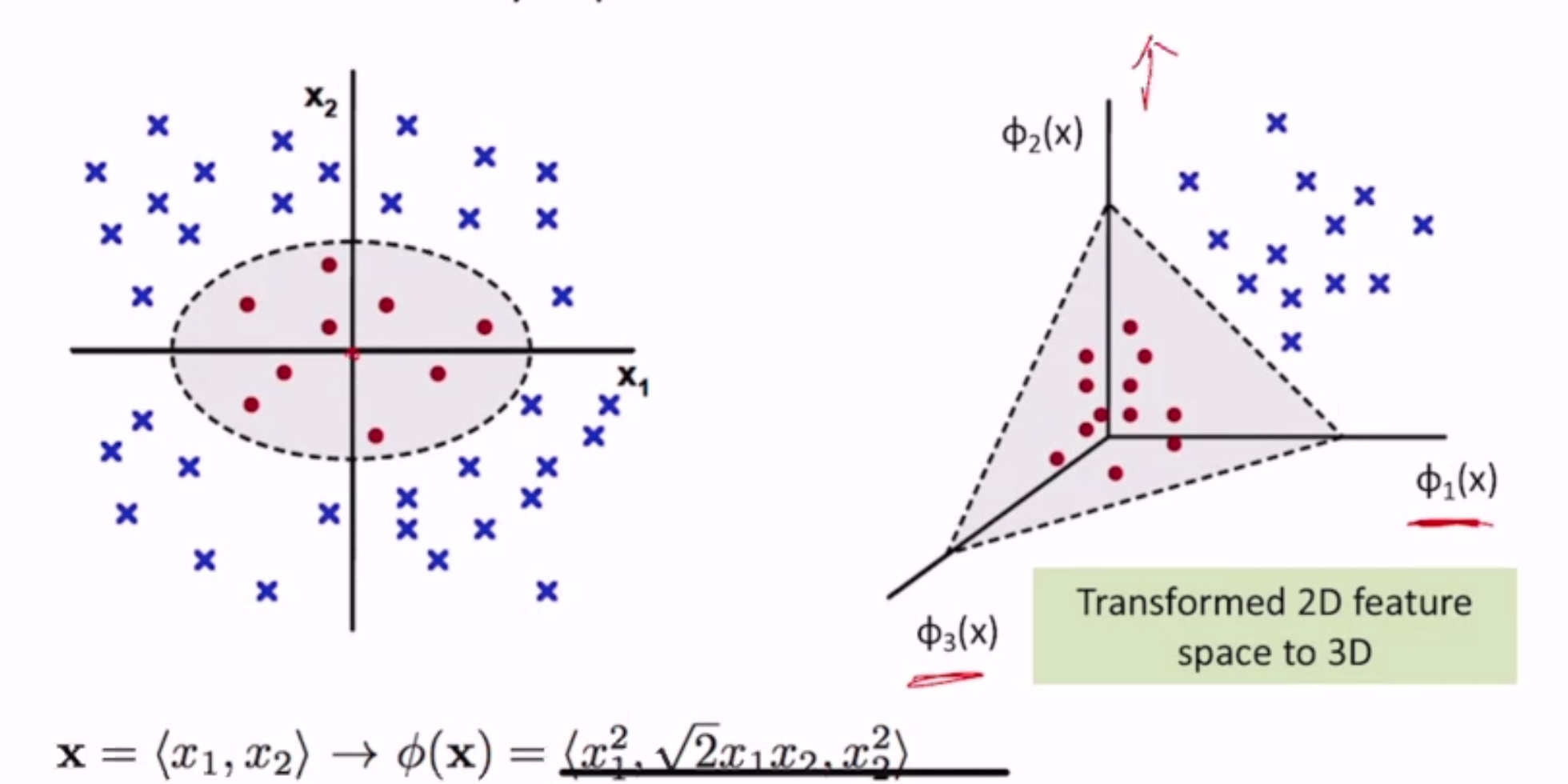### Feature Mapping¶

We can map the original feature vector to a higher dimensional space $$\phi(x)$$

$\begin{split}\phi(x) = < & 1, 2x_1, 2x_2, ..., 2x_D, \\ & x_1^2, x_1x_2, ..., x_1x_D, \\ & x_2x_1, x_2^2, ..., x_2x_D, \\ & ... >\end{split}$